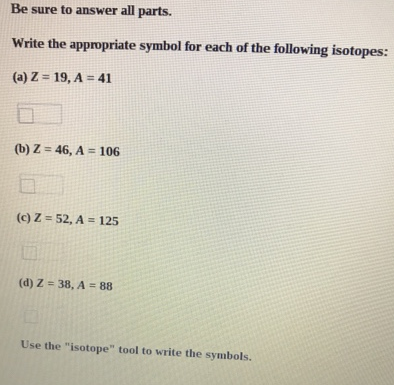# Problem: Be sure to answer all parts.Write the appropriate symbol for each of the following isotopes: (a) Z = 19, A = 41 (b) Z = 46, A = 106 (c) Z = 52, A = 125 (d) Z = 38, A = 88 Use the "isotope" tool to write the symbols.

###### FREE Expert Solution
92% (174 ratings)###### Problem Details

Be sure to answer all parts.

Write the appropriate symbol for each of the following isotopes:

(a) Z = 19, A = 41

(b) Z = 46, A = 106

(c) Z = 52, A = 125

(d) Z = 38, A = 88

Use the "isotope" tool to write the symbols.